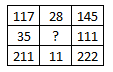Question 16

# Select the missing number from the given responses ?Solution

In the first row, the number at the end is obtained by adding the other 2

Eg = $$117+28=145$$

and $$211+11=222$$

Similarly, $$35+x=111$$

=> $$x=111-35=76$$

=> Ans - (B)